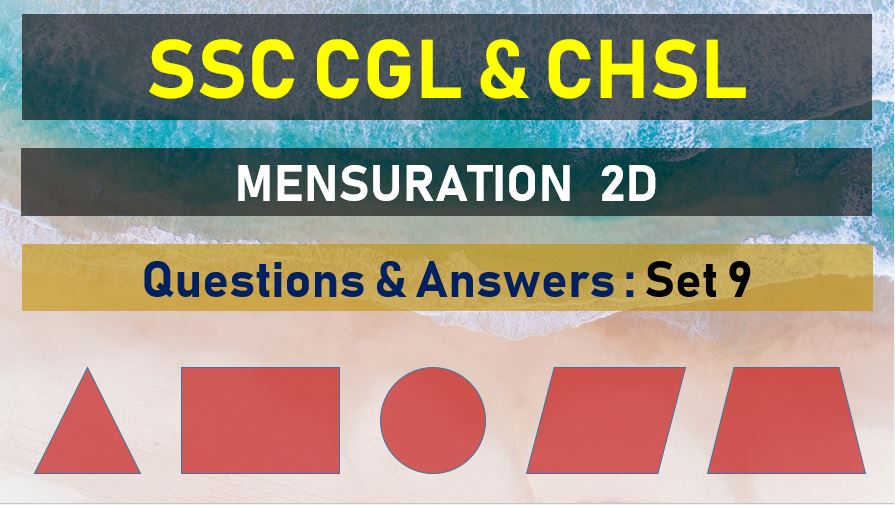Friday, May 7, 2021
Home > mensuration > SSC CGL Area and Perimeter Questions Answers Set 9

# SSC CGL Area and Perimeter Questions Answers Set 9SSC Mensurations 2D
Area and Perimeter Questions Sets
Set 1Set 2Set 3Set 4Set 5
Set 6Set 7Set 8Set 9Set 10

SSC CGL Area and Perimeter Questions Answers Set 9: Ques No 1

The area of circle whose radius is 6 cm is trisected by two concentric circles. The radius of the smallest circle is

Options:

A. 2√3 cm

B. 2√6 cm

C. 2 cm

D. 3 cm

Solution:

SSC CGL Area and Perimeter Questions Answers Set 9: Ques No 2

The area of an equilateral triangle inscribed in a circle is 4√3 cm2. The area of the circle is

Options:

A. 16/3 π cm2

B. 22/3 π cm2

C. 28/3 π cm2

D. 42/3 π cm2

Solution:

SSC CGL Area and Perimeter Questions Answers Set 9: Ques No 3

If the difference between the circumference and diameter of a circle is 30 cm, then the radius of the
circle must be

Options:

A. 6 cm

B. 7 cm

C. 8 cm

D. 5 cm

Solution:

SSC CGL Area and Perimeter Questions Answers Set 9: Ques No 4

The base and altitude of a right angled triangle are 12 cm and 5 cm respectively. The perpendicular distance of its hypotenuse from the opposite vertex is

Options:

A. 56/13 cm

B. 60/13 cm

C. 5 cm

D. 7 cm

Solution:

SSC CGL Area and Perimeter Questions Answer Set 9: Ques No 5

The area of a circle is increased by 22 cm, if its radius is increased by 1 cm . the original radius of circle is

Options:

A. 6 cm

B. 3.2 cm

C. 3 cm

D. 3.5 cm

Solution:

SSC CGL Area and Perimeter Questions Answer Set 9: Ques No 6

The area of the largest circle, that can be drawn inside a rectangle with sides 148 cm . by 14 cm is

Options:

A. 49 cm2

B. 154 cm2

C. 378 cm2

D. 1078 cm2

Solution:

SSC CGL Area and Perimeter Questions Answer Set 9: Ques No 7

A circle is inscribed in an equilateral triangle of side 8 cm. The area of the portion between the triangle and the circle is

Options:

A. 11 cm2

B. 10.95 cm2

C. 10 cm2

D. 10.50 cm2

Solution:

SSC CGL Area and Perimeter Questions Answer Set 9: Ques No 8

In a triangular field having sides 30 m, 72 m and 78 m, the length of the altitude to the side measuring 72 m is

Options:

A. 25 cm

B. 28 cm

C. 30 cm

D. 35 cm

Solution:

SSC CGL Area and Perimeter Questions Answer Set 9: Ques No 9

If the perimeter of a right-angled isosceles triangle is (4√2 +4) cm,the length of the hypotenuse is

Options:

A. 4 cm

B. 6 cm

C. 8 cm

D. 10 cm

Solution:

SSC CGL Area and Perimeter Questions Answer Set 9: Ques No 10

ABC is an equilateral triangle of side 2 cm with A, B, C as center and radius 1 cm, three arcs are drawn. The area of the region with in the triangle bounded by the three arcs is

Options:

A. (3√3 – π/2)

B. (√3 – 3π/2)

C. (√3 – π/2)

D. (π/2 – √3)

Solution:

SSC CGL Area and Perimeter Questions Answer Set 9: Ques No 11

The ratio between the length and the breadth of a rectangular park is 3 : 2. If a man cycling along the boundary of the park at the speed of 12 km/hr completes one round in 8 minutes, then the area of the park (in sq. m) is:

Options:

A. 15360

B. 153600

C. 30720

D. 307200